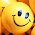### Buying a car - Car loan or full cash payment?

Planning to buy a new car? First step is buying a car is deciding the model. There are many Indian and foreign car makers launching 2-3 new models every year. So finalizing a model is not an easy task. Get advise from friends and relatives, read online review, if time permits, take test drives.

Once the model is decided, next barrier is payment. Now most of the private and public banks offers up to 85% of the total amount as loan, that also at attractive rates. Also some accumulate cash over time to fulfill their wish, they prefer to go for full cash payment, mainly to avoid paper work and the interest payment.

In this article, we are doing some rough mathematics to see how much you would be overpaying if you are taking loan instead of cash payment. As we mentioned, it is a rough calculation, not all factors are covered.

Suppose the cost of the car you are planning to buy is Rs. 5,00,000(5L) and you are availing a loan of Rs. 1,00,000(1L).

So the EMI with interest rate of 10.5% for a repayment period of 36 months would be around Rs. 13,001. In 3 years you will repay Rs. 4,68,036 (36 x 13,001). So, at the end of 3 years, total amount spent on your car would be around Rs. 5,68,036. Let's consider the interest lost on the EMI paid for three years, it will come around Rs. 64,401, so total expense would be Rs. 6,32,437.

Suppose you have Rs. 4,00,000 cash in hand but put it in a fixed deposit scheme with an interest rate of 8% and then going for loan. You will be earning an interest of approximately Rs. 1,01,044 over 3 years period  for Rs. 4L you deposited. So, let's deduct the interest you earned from the expense, Rs. 4,68,036 - Rs. 1,01,044 = Rs. 3,66,992. So, total expense would be Rs. 5,31,393 if you keep 4L in FD and take 4L loan.

1L Cash & 4L loan: Total expense(after 3 years): 1L(down payment) + Rs. 4,68,036(EMI paid) - Rs. 1,01,044(interest earned from FD)  + Rs. 64,401 (Interest lost on EMI paid) = Rs. 5,31,393

If you have paid full amount during the purchase, the calculation would be:

Cash payment: Total expense(after 3 years):  1,00,000 + 4,00,000 + (Interest lost on 4L = 1,01,044) = Rs. 6,01,044

So, what is the conclusion? You can save some amount if you go for car loan! Interesting, isn't it? If you disagree with the calculation provided, feel free to post it in the comments section.

Disclaimer: This is just for illustration purpose only, please check with your bank for the latest interest rate and other terms and conditions.

1.It is the way you calculate it.
You assume that you have ~4.2 L in your account to earn interest for 3 years. This is not true since you will be making repayments every month and your principal will decrease and hence the interest you earn each month will also go down. Not to mention the interest earned will be taxable.

2.Its true that your interest is on reducing principal, but the EMI is fixed and what calculation he has given is correct. The funda is go for car loan definitely if you are buying new car. Go for cash if you are buying a second hand pieceas the rate of interest is around 15%.

Cheers!!!

3.Yes, the principal will reduce over time. In addition, you haven't included tax payments on the FD that you suggest, so interest earned will be considerably less in actuality. Besides how can you earn more from a 6% interest rate on deposit when paying over 10% on the loan?

4.Thank you for the meaningful and useful service that you have shared with us above.Really it is worthy to me and seems to me very helpful.

5.6.Hi people,
Thank you so much for this wonderful article really!